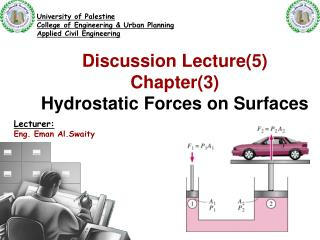DownloadDownload PresentationDiscussion Lecture(5) Chapter(3) Hydrostatic Forces on Surfaces

# Discussion Lecture(5) Chapter(3) Hydrostatic Forces on Surfaces

Download Presentation## Discussion Lecture(5) Chapter(3) Hydrostatic Forces on Surfaces

- - - - - - - - - - - - - - - - - - - - - - - - - - - E N D - - - - - - - - - - - - - - - - - - - - - - - - - - -
##### Presentation Transcript

1. University of Palestine College of Engineering & Urban Planning Applied Civil Engineering Discussion Lecture(5) Chapter(3) Hydrostatic Forces on Surfaces Lecturer: Eng. Eman Al.Swaity

2. 3.4 HORIZONTAL PLANE SURFACE SUBMERGED IN LIQUID Total pressure is defined as the force exerted by a static fluid on a surface either plane or curved when the fluid comes in contact with the surfaces. This force always acts normal to the surface Centre of pressure is defined as the point of application of the total pressure on the surface. There are four cases of submerged surfaces on which the total pressure force and centre of pressure is to be determined. The submerged surfaces maybe : 1. Vertical plane surface, 2. Horizontal plane surface, 3. Inclined plane surface, and 4. Curved surface.

3. 3.4 HORIZONTAL PLANE SURFACE SUBMERGED IN LIQUID

4. Problem 3.13 Fig. shows a tank full of water. Find :(i) Total pressure on the bottom of tank. (ii) Weight of water in the tank. (iii) Hydrostatic paradox between the results of (i) and (ii). Width of tank is 2 m.

5. Problem 3.13-solution

6. 3.5 INCLINED PLANE SURFACE SUBMERGED IN LIQUID

7. Problem 3.14 A rectangular plane surface 3 m wide and 4 m deep lies in water in such a way that its plane makes an angle of 30° with the free surface of water. Determine the total pressure force and position of centre of pressure, when the upper edge is 2 m below the free surface.

8. Problem 3.14-solution

9. Problem 3.14-solution

10. Problem 3.15 A circular plate 3.0 m diameter is immersed in water in such a way that its greatest and least depth below the free surface are 4 m and 1.5 m respectively. Determine the total pressure on one face of the plate and position of the centre of pressure.

11. Problem 3.15-solution

12. Problem 3.15-solution

13. Problem 3.17 A rectangular gate 5m.x2m.is hinged at its base and inclined at 60° to the horizon­tal as shown in Fig. 3.22. To keep the gate in a stable position, a counter weight of 5000 kgf is attached at the upper end of the gate as shown in figure. Find the depth of water at which the gate begins to fall. Neglect the weight of the gate and friction at the hinge and pulley.

14. Problem 3.17-solution

15. Problem 3.17-solution

16. Problem 3.17-solution

17. End of the Lecture Let Learning Continue